Courses

# Ex 1.3 NCERT Solutions - Number System Class 9 Notes | EduRev

## Class 9 Mathematics by VP Classes

Created by: Vp Classes

## Class 9 : Ex 1.3 NCERT Solutions - Number System Class 9 Notes | EduRev

The document Ex 1.3 NCERT Solutions - Number System Class 9 Notes | EduRev is a part of the Class 9 Course Class 9 Mathematics by VP Classes.
All you need of Class 9 at this link: Class 9

Q.1. Write the following in decimal form and say what kind of decimal expansion each has:
(i) 36/100
(ii) 1/11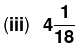(iv) 3/13
(v) 2/11
(vi) 329/400
Solution.
(i) We have 36/100 = 0.36
∴ The decimal expansion of 36/100 is terminating.
(ii) Dividing 1 by 11, we have: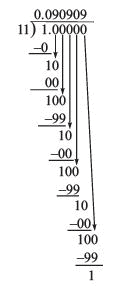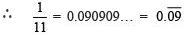Thus, the decimal expansion is “non-terminating repeating”.

Note: The bar above the digits indicates the block of digits that repeats. Here, the repeating block is 09.

(iii) To write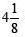in p/q from, we have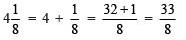Now, dividing 33 by 8, we have :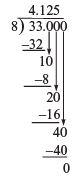Remainder = 0, means the process of division terminates.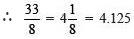Thus, the decimal expansion is terminating.

(iv) Dividing 3 by 13, we have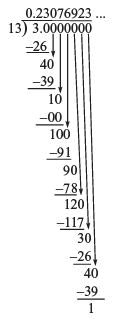Here, the repeating block of digits is 230769.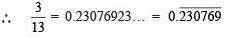Thus, the decimal expansion of 3/13  is “non-terminating repeating”.

(v) Dividing 2 by 11, we have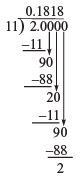Here, the repeating block of digits is 18.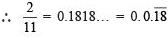Thus, the decimal expansion of 2/11 is “non-terminating repeating”.

(vi) Dividing 329 by 400, we have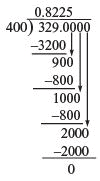Remainder = 0, means the process of division terminates.
∴ 329/400 = 0.8225
Thus, the decimal expansion of 329/400  is terminating.

Q.2. You know that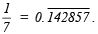Can you predict what the decimal expansions of  2/7, 3/7, 4/7, 5/7, 6/7 are, without actually doing the long division? If so, how?
Solution.
We are given that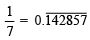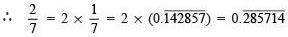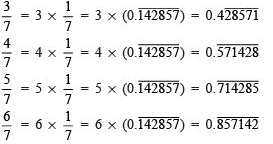Thus, without actually doing the long division we can predict the decimal expansions of the above given rational numbers.

Q.3. Express the following in the form (p\q), where p and q are integers and q ≠ 0.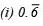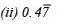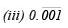Solution.
(i) Let x =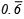= 0.6666…
Since, there is one repeating digit.
∴  We multiply both sides by 10,
10x = (0.666…) x 10
or 10x = 6.6666…
∴ 10x - x = 6.6666... - 0.6666...
or 9x = 6
or x = 6/9 = 2/3
Thus,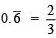(ii)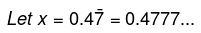∴ 10x = 10 x (0.4777...)
or   10x = 4.777       ...(1)
and  100x = 47.777   ...(2)
Subtracting (1) from (2), we have
100x – 10x = (47.777…) – (4.777…)
90x = 43
or x = 43/90
Thus,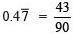(iii)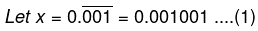Here, we have three repeating digits after the decimal point, therefore we multiply by 1000.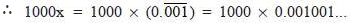or   1000x = 1.001001   …...(2)
Subtracting (1) from (2), we have
1000x – x = (1.001…) – (0.001…)
or 999x = 1
∴ x = 1/999
Thus,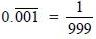Q.4. Express 0.99999… in the form p/q . Are you surprised by your answer? With your teacher and classmates discuss why the answer makes sense.
Solution.

Let x = 0.99999  .....(1)
Multiply both sides by 10,
we have [∵ There is only one repeating digit.]
10 x x = 10 x (0.99999…)
or 10x = 9.9999      ...(2)
Subtracting (1) from (2),
we get 10x – x = (9.9999…) – (0.9999…)
or 9x = 9
or x = 9/9 = 1
Thus, 0.9999… = 1 As 0.9999… goes on forever, there is no gap between 1 and 0.9999
Hence both are equal.

Q.5. What can the maximum number of digits be in the repeating block of digits in the decimal expansion of (1/17)? Perform the division to check your answer.
Solution.
Since, the number of entries in the repeating block of digits is less than the divisor.
In 1/17, the divisor is 17.
∴  The maximum number of digits in the repeating block is 16. To perform the long division, we have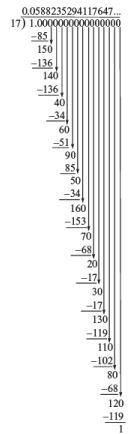The remainder 1 is the same digit from which we started the division.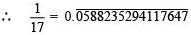Thus, there are 16 digits in the repeating block in the decimal expansion of 1/17. Hence, our answer is verified.

Q.6. Look at several examples of rational numbers in the form p/q  (q ≠ 0), where p and q are integers with no common factors other than 1 and having terminating decimal representations (expansions). Can you guess what property q must satisfy?
Solution:
Let us look at decimal expansion of the following terminating rational numbers: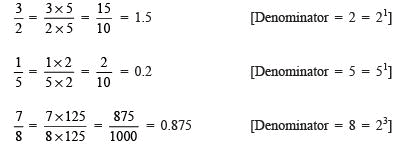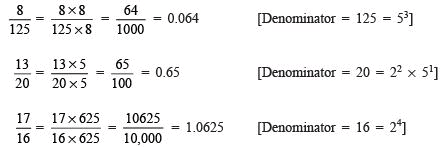We observe that the prime factorization of q (i.e. denominator) has only powers of 2 or powers of 5 or powers of both.

Note: If the denominator of a rational number (in its standard form) has prime factors either 2 or 5 or both, then and only then it can be represented as a terminating decimal.

Q.7. Write three numbers whose decimal expansions are non-terminating non-recurring.
Solution.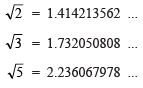Q.8. Find three different irrational numbers between the rational numbers (5/7) and (9\11).
Solution. To express decimal expansion of 5/7 and 9/11, we have: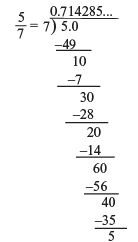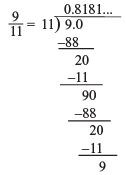As there are an infinite number of irrational numbers between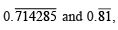any three of them can be:
(i) 0.750750075000750…
(ii) 0.767076700767000767…
(iii) 0.78080078008000780…

Q.9. Classify the following numbers as rational or irrational:
(i)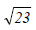(ii)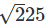(iii) 0.3796
(iv) 7.478478
(v) 1.101001000100001…
Solution.
(i) ∵ 23 is not a perfect square.
∴  is an irrational number.

(ii)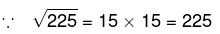∴ 225 is a perfect square.
Thus, 225 is a rational number.

(iii) ∵ 0.3796 is a terminating decimal,
∴ It is a rational number.

(iv) 7.478478… =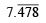Since,is a non-terminating and recurring (repeating) decimal.
∴ It is a rational number.

(v) Since, 1.101001000100001… is a non-terminating and non-repeating decimal number.
∴ It is an irrational number.

REMEMBER
A rational number is a number we can know exactly, either as a whole number, a fraction or a mixed number, but not always exactly as a decimal.
An irrational number can never be known in any form.

Offer running on EduRev: Apply code STAYHOME200 to get INR 200 off on our premium plan EduRev Infinity!

132 docs

,

,

,

,

,

,

,

,

,

,

,

,

,

,

,

,

,

,

,

,

,

;